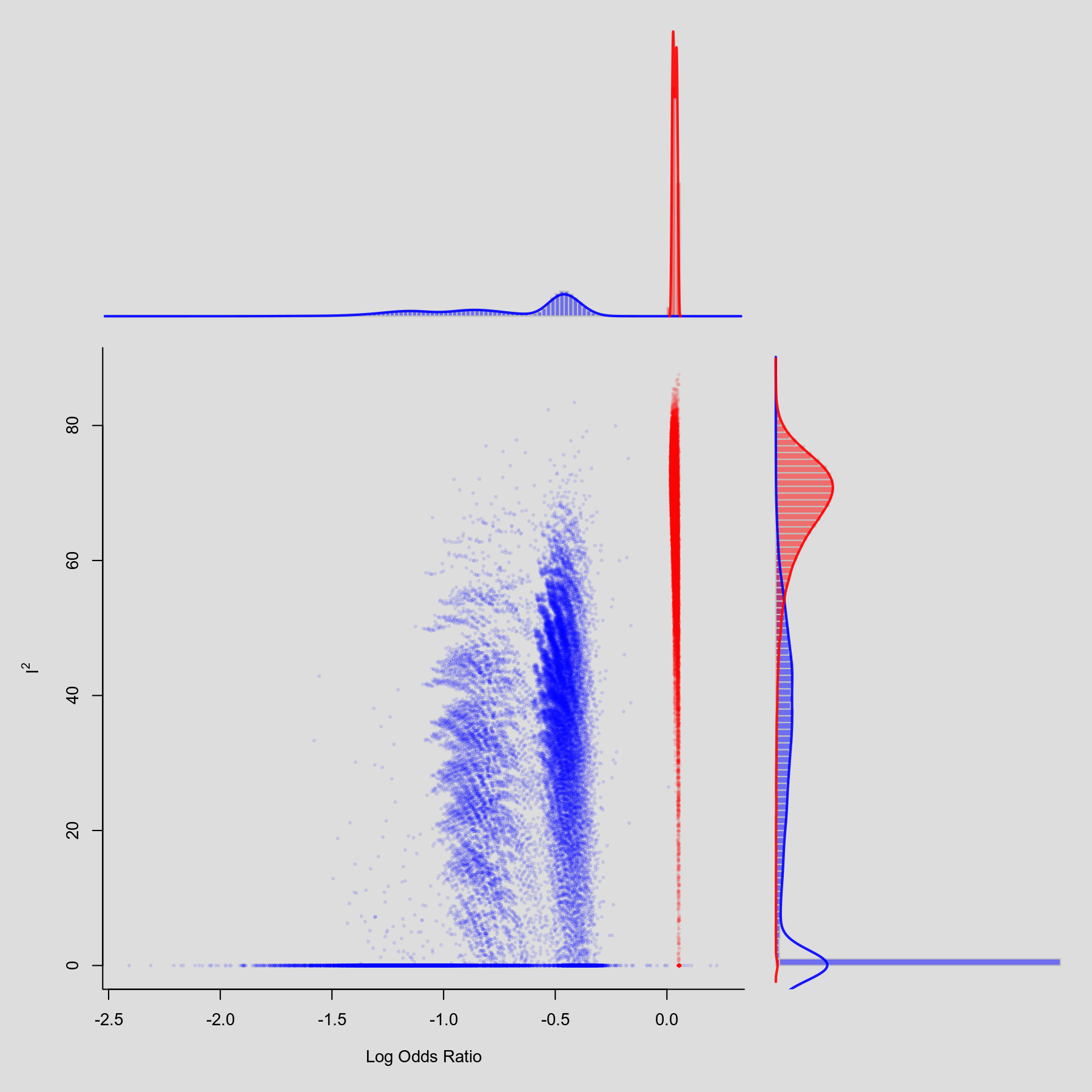#The metafor Package

A Meta-Analysis Package for R

plots:gosh_plot

## GOSH Plot

### Description

Olkin, Dahabreh, and Trikalinos (2012) proposed the GOSH (graphical display of study heterogeneity) plot, which is based on examining the results of an equal-effects model in all possible subsets of size $1, \ldots, k$ of the $k$ studies included in a meta-analysis. In a homogeneous set of studies, the model estimates obtained this way should form a roughly symmetric, contiguous, and unimodal distribution. On the other hand, when the distribution is multimodal, then this suggests the presence of heterogeneity, possibly due to outliers and/or distinct subgroupings of studies. Plotting the estimates against some measure of heterogeneity (e.g., $I^2$, $H^2$, or the Q-statistic) can also help to reveal subclusters, which are indicative of heterogeneity.

A nice example to illustrate the method is the meta-analysis of trials that examined the effectiveness of intravenous magnesium in the prevention of death following acute myocardial infarction (data from Egger et al., 2001). While the smaller studies suggested that magnesium is an effective treatment for reducing mortality, the results from the ISIS-4 mega trial (ISIS-4 Collaborative Group, 1995) indicated no reduction in mortality with magnesium treatment.

Below, an equal-effects model is first fitted to all trials and then the gosh() function is used to fit equal-effects models in all possible subsets. The GOSH plot is then created by plotting the results. The points for subsets that include the ISIS-4 trial (study 16 in the dataset) are shown in red and blue otherwise.

### Plot### Code

library(metafor)

### meta-analysis of all trials including ISIS-4 using an equal-effects model
res <- rma(measure="OR", ai=ai, n1i=n1i, ci=ci, n2i=n2i, data=dat.egger2001, method="EE")

### fit EE model to all possible subsets
sav <- gosh(res)

### create GOSH plot
### red points for subsets that include and blue points
### for subsets that exclude study 16 (the ISIS-4 trial)
plot(sav, out=16, breaks=100)

Egger, M., Davey Smith, G., & Altman, D. G. (Eds.) (2001). Systematic reviews in health care: Meta-analysis in context (2nd ed.). London: BMJ Books.

ISIS-4 Collaborative Group (1995). ISIS-4: A randomised factorial trial assessing early oral captopril, oral mononitrate, and intravenous magnesium sulphate in 58,050 patients with suspected acute myocardial infarction. Lancet, 345, 669–685.

Olkin, I., Dahabreh, I. J., & Trikalinos, T. A. (2012). GOSH - a graphical display of study heterogeneity. Research Synthesis Methods, 3(3), 214–223.